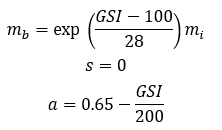top of page

# P-y curve model of massive rock - Liang et al. (2009)

P-Y curve model of massive rock is proposed by Liang et al. (2009). This model calculates the ultimate lateral resistance of rock with considering both a wedge failure at shallow depths and a pile shear/compression failure at deeper depths, as shown in the figure below.This p-y curve model is incorporated into the PileLAT and PileGroup programs and shown in the figure below.1.0 Calculation of ultimate lateral resistance at shallow depth

The ultimate lateral resistance of massive rock at the shallow depth is given by the following equations:where c is the effective cohesion, Phi' is the effective friction angle, Gamma is the effective unit weight and D is the pile diameter/width.

2.0 Calculation of ultimate lateral resistance at deep depth

The ultimate lateral resistance of massive rock at the deep depth is given by the following equations:where Sigma-V' is the effective overburden pressure at the deep depth and Sigma-c is the unconfined compressive strength of rock mass. The lesser of those ultimate resistance values (shallow and deep depths) will be adopted in the analysis.

The initial slope of the P-Y curve, Ki can be determined by the following equation:Where Em is the rock mass modulus, D is the pile diameter, EpIp is the bending stiffness of the pile, Dref is the reference pile diameter which is equal to 0.305 m and mu is Poisson’s ratio of the pile.

The effective strength parameters of massive rock, c' and Phi' are determined using the Hoek-Brown strength criterion as follows in the program:where mb , a and s are material constants of rock and determined by the following method in PileLAT.

For GSI>25, which represents rock masses of good to reasonable qualityFor GSI<25, which represents rock masses of very poor quality3.0 Massive rock p-y curve model parameters

The required input parameters for massive rock model in the PileLAT program are summarized as below:

• Total Unit Weight: The total unit weight of the soil material

• UCS: Unconfined compressive strength (kPa)

• UCS Strength – Rate of change: The increase rate of the unconfined compressive strength (UCS) with the depth from the top of the soil layer

• Elastic Modulus, Em: Elastic rock mass modulus at the top of the layer top

• Modulus, Rate of Change, Em-inc: It is the rock mass modulus increment rate with the layer depth from the top of the rock layer

• Material Constant, Mi: Intact rock constant which depends on the rock type and normally ranges from 4 to 33

• GSI: Geological strength indexThe typical values for rock material constant (mi) can be opened by the users by clicking the "..." button next to the input field of material constant. The following figure shows the dialog of typical rock material constant values. This dialog helps the users to select the correct value based on the rock type.The typical values for rock geological strength index (GSI) can be opened by the users by clicking the "..." button next to the input field of GSI. The following figure shows the dialog of typical rock geological strength index values. This dialog helps the users to select the correct value based on the rock type.4.0 Typical p-y curves for massive rock model

Typical p-y curves for massive rock model in the PileLAT program are shown in the figure below.5.0 Some notes on massive rock p-y curve model for laterally loaded piles

Note that it is likely more deflection and lower lateral resistance will be estimated when the GSI values are less than 30 when massive rock p-y curve model is used. This is due to the underlying assumptions on the empirical relationships based on Hoek-Brown method, where rock mass strength and mass modulus exponentially reduces with lower GSI values.

The limitations of Hoek-Brown relationships in low strength/ low GSI materials are also applicable to massive rock p-y curve model. It is well-known that the original Hoek-Brown criterion was developed using testing data with UCS greater than 15 MPa. All the six tests adopted by Liang et al. (2009) have intact UCS greater than 15 MPa.

Sarath and Marco (2023) proposed the following interim recommendations on the use of massive rock p-y curve model based on the intact UCS strength.Reference:

Liang, R., Yang. K., and Nusairat, J. (2009). “p-y Criterion for Rock Mass”, Journal of Geotechnical and Geoenvironmental Engineering, Vol. 135, No. 1, pp. 26-36.

Sarath Somasundaram and Marco Rafanelli (2023). "Laterally loaded rock socket design - A review of the P-y curve design approach", Proceedings of the 14th Australia and New Zealand Conference on Geomechanics, Cairns 2023 (ANZ2023).

180 views